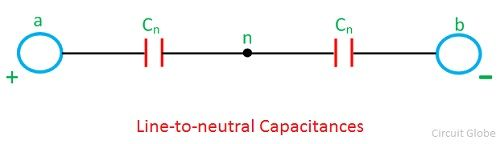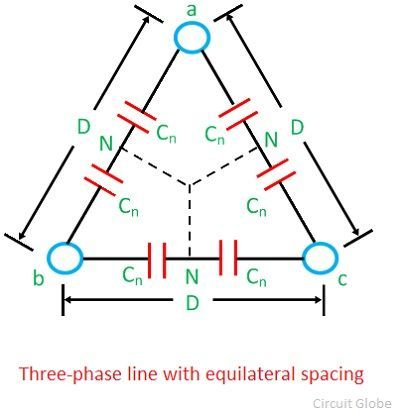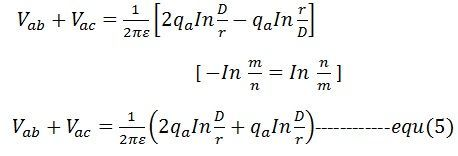# Capacitance of Transmission Line

Transmission line conductors constitute a capacitor between them. The conductors of the transmission line act as a parallel plate of the capacitor and the air is just like a dielectric medium between them. The capacitance of a line gives rise to the leading current between the conductors. It depends on the length of the conductor.

The capacitance of the line is proportional to the length of the transmission line. Their effect is negligible on the performance of short (having a length less than 80 km) and low voltage transmission line. In the case of high voltage and long lines, it is considered as one of the most important parameters.

### Capacitance of two-wire line

The capacitance of the transmission line along with the conductances forms the shunt admittance. The conductance in the transmission line is because of the leakage over the surface of the conductor. Considered a line consisting of two conductors a and b each of radius r. The distance between the conductors being D shown in the diagram below:-The potential difference between the conductors a and b isWhere, qa – charge on conductor a
qb – charge on conductor b
Vab – potential difference between conductor a and b
ε- absolute permittivitySubstituting these values in voltage equation we get,The capacitance between the conductors isCab is referred to as line-to-line-capacitance.

If the two conductors a and b are oppositely charged, and the potential difference between them is zero, then the potential of each conductor is given by 1/2 Vab.The capacitance between each conductor and point of zero potential n isCapacitance Cn is called the capacitance to neutral or capacitance to ground.

Capacitance Cab is the combination of two equal capacitance a and b in series. Thus, capacitance to neutral is twice the capacitance between the conductors, i.e.,The absolute permittivity ε is given bywhere εo is the permittivity of the free space and εr is the relative permittivity of the medium.Capacitance reactance between one conductor and neutral

###Capacitance of the symmetrical three-phase line

Let a balanced system of voltage be applied to a symmetrical three-phase-line shown belowThe phasor diagram of the three-phase line with equilateral spacing is shown below:Take the voltage of conductor a to neutral as a reference phasorThe potential difference between conductor a and b can be written asThe capacitance of symmetrical three-phase line is same as that of the two-wire line.

### 3 thoughts on “Capacitance of Transmission Line”

1. Soubhik Bhattacharya

Then what is the line to line capacitance in 3-phase?
Is it the delta equivalent capacitances determined from line to neutral capacitances?

2. A very informative/ educative and useful piece, supported by relevant mathematical equations.